# NCERT Solutions For Class 11 physics Mechanical Properties of Solids## myCBSEguide App

CBSE, NCERT, JEE Main, NEET-UG, NDA, Exam Papers, Question Bank, NCERT Solutions, Exemplars, Revision Notes, Free Videos, MCQ Tests & more.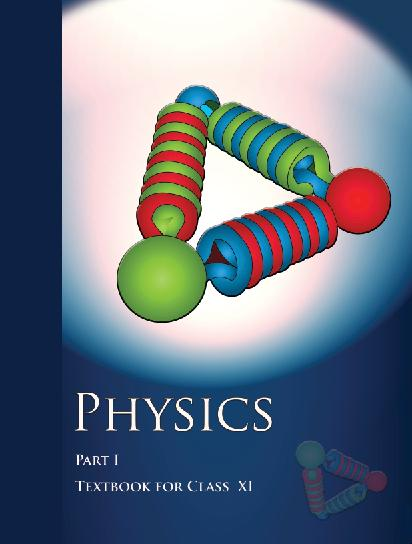## NCERT Class 11 Physics Chapter wise Solutions

• 1 – Physical World
• 2 – Units and Measurements
• 3 – Motion in a Straight line
• 4 – Motion in a Plane
• 5 – Laws of Motion
• 6 – Work, Energy and Power
• 7 – System of Particles and Rotational motion
• 8 – Gravitation
• 9 – Mechanical Properties of Solids
• 10 – Mechanical properties of fluids
• 11 – Thermal Properties of matter
• 12 – Thermodynamics
• 13 – Kinetic Theory
• 14 – Oscillations
• 15 – Waves

## CHAPTER 9 MECHANICAL PROPERTIES OF SOLIDS

• 9.1 Introduction
• 9.2 Elastic behaviour of solids
• 9.3 Stress and strain
• 9.4 Hooke’s law
• 9.5 Stress-strain curve
• 9.6 Elastic moduli
• 9.7 Applications of elastic behaviour of materials

## NCERT Solutions For Class 11 physics Mechanical Properties of Solids

1. A steel wire of length 4.7 m and cross-sectional area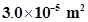stretches by the same amount as a copper wire of length 3.5 m and cross-sectional area of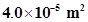under a given load. What is the ratio of the Young’s modulus of steel to that of copper?

2. Figure 9.11 shows the strain-stress curve for a given material. What are (a) Young’s modulus and (b) approximate yield strength for this material?

3. The stress-strain graphs for materials A and B are shown in Fig. 9.12.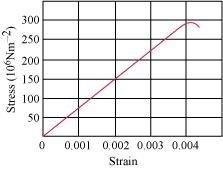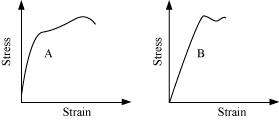The graphs are drawn to the same scale.

(a) Which of the materials has the greater Young’s modulus?

(b) Which of the two is the stronger material?

4. Read the following two statements below carefully and state, with reasons, if it is true or false.

(a) The Young’s modulus of rubber is greater than that of steel;

(b) The stretching of a coil is determined by its shear modulus.

5. Two wires of diameter 0.25 cm, one made of steel and the other made of brass are loaded as shown in Fig. 9.13. The unloaded length of steel wire is 1.5 m and that of brass wire is 1.0 m. Compute the elongations of the steel and the brass wires.

6. The edge of an aluminum cube is 10 cm long. One face of the cube is firmly fixed to a vertical wall. A mass of 100 kg is then attached to the opposite face of the cube. The shear modulus of aluminium is 25 GPa. What is the vertical deflection of this face?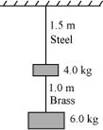7. Four identical hollow cylindrical columns of mild steel support a big structure of mass 50,000 kg. The inner and outer radii of each column are 30 cm and 60 cm respectively. Assuming the load distribution to be uniform, calculate the compressional strain of each column.

8. A piece of copper having a rectangular cross-section of 15.2 mm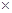19.1 mm is pulled intension with 44,500 N force, producing only elastic deformation. Calculate the resulting strain?

9. A steel cable with a radius of 1.5 cm supports a chairlift at a ski area. If the maximum stress is not to exceed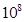N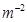, what is the maximum load the cable can support?

10. A rigid bar of mass 15 kg is supported symmetrically by three wires each 2.0 m long. Those at each end are of copper and the middle one is of iron. Determine the ratio of their diameters if each is to have the same tension.

11. A 14.5 kg mass, fastened to the end of a steel wire of unstretched length 1.0 m, is whirled in a vertical circle with an angular velocity of 2 rev/s at the bottom of the circle. The cross-sectional area of the wire is 0.065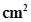. Calculate the elongation of the wire when the mass is at the lowest point of its path.

12. Compute the bulk modulus of water from the following data: Initial volume = 100.0 litre, Pressure increase = 100.0 atm (1 atm =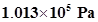), Final volume = 100.5 litre. Compare the bulk modulus of water with that of air (at constant temperature). Explain in simple terms why the ratio is so large.

13. What is the density of water at a depth where pressure is 80.0 atm, given that its density at the surface is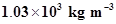?

14. Compute the fractional change in volume of a glass slab, when subjected to a hydraulic pressure of 10 atm.

15. Determine the volume contraction of a solid copper cube, 10 cm on an edge, when subjected to a hydraulic pressure of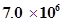Pa.

16. How much should the pressure on a litre of water be changed to compress it by 0.10%?

17. Anvils made of single crystals of diamond, with the shape as shown in Fig. 9.14, are used to investigate behaviour of materials under very high pressures. Flat faces at the narrow end of the anvil have a diameter of 0.50 mm, and the wide ends are subjected to a compressional force of 50,000 N. What is the pressure at the tip of the anvil?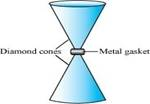18. A rod of length 1.05 m having negligible mass is supported at its ends by two wires of steel (wire A) and aluminium (wire B) of equal lengths as shown in Fig. 9.15. The cross-sectional areas of wires A and B are 1.0 mm2 and 2.0 mm2, respectively. At what point along the rod should a mass m be suspended in order to produce (a) equal stresses and (b) equal strains in both steel and aluminium wires.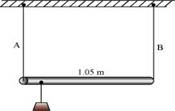19. A mild steel wire of length 1.0 m and cross-sectional area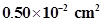is stretched, well within its elastic limit, horizontally between two pillars. A mass of 100 g is suspended from the mid-point of the wire. Calculate the depression at the midpoint.

20. Two strips of metal are riveted together at their ends by four rivets, each of diameter 6.0 mm. What is the maximum tension that can be exerted by the riveted strip if the shearing stress on the rivet is not to exceed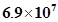Pa? Assume that each rivet is to carry one quarter of the load.

21. The Marina trench is located in the Pacific Ocean, and at one place it is nearly eleven km beneath the surface of water. The water pressure at the bottom of the trench is about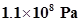. A steel ball of initial volume 0.32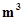bis dropped into the ocean and falls to the bottom of the trench. What is the change in the volume of the ball when it reaches to the bottom?

## NCERT Solutions for Class 11 Physics

NCERT Solutions Class 11 Physics PDF (Download) Free from myCBSEguide app and myCBSEguide website. Ncert solution class 11 physics includes text book solutions from both part 1 and part 2. NCERT Solutions for CBSE Class 11 Physics have total 15 chapters. 11 Physics NCERT Solutions in PDF for free Download on our website. Ncert physics class 11 solutions PDF and physics ncert class 11 PDF solutions with latest modifications and as per the latest CBSE syllabus are only available in myCBSEguide

## CBSE app for Class 11

To download NCERT Solutions for class 11 Physics, Chemistry, Biology, History, Political Science, Economics, Geography, Computer Science, Home Science, Accountancy, Business Studies and Home Science; do check myCBSEguide app or website. myCBSEguide provides sample papers with solution, test papers for chapter-wise practice, NCERT solutions, NCERT Exemplar solutions, quick revision notes for ready reference, CBSE guess papers and CBSE important question papers. Sample Paper all are made available through the best app for CBSE students and myCBSEguide website